﻿ Digital Circuits and Systems - Circuits i Sistemes Digitals (CSD) - EETAC - UPCBachelor's Degree in Telecommunications Systems and in Network EngineeringLecture 1 L2.1: Designing standard logic circuits using VHDL. Multiplexers [P2] Concepts associated to standard logic circuits. [28/2]

1.7. Standard combinational logic circuits

Once we have understood P1 basic ideas on Boole's algebra and circuit analysis and design using logic gates, let us continue presenting basic standard combinational blocks.

1.7.1. Concept map

All combinational circuits (including arithmetic circuits in and ) are organised following this concept map in Fig. 1 and Fig. 2

1.7.1.1. Truth table, symbol, timing diagram

1.7.1.2. Chip expansion, enable input

1.7.1.3. Examples of commercial chips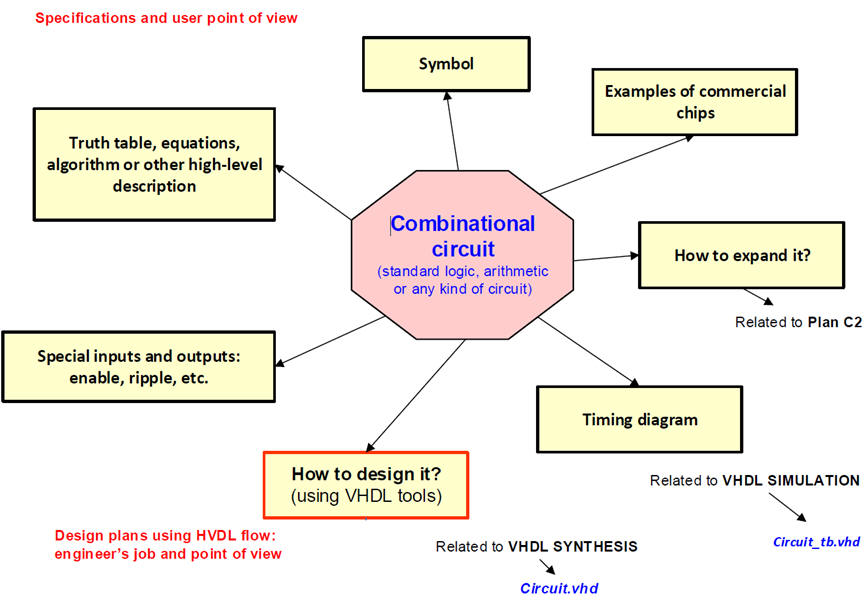Fig. 1. Concept map for organising circuit specifications. (Visio)

1.7.1.4. VHDL design plan (our list of design )Fig. 2. The three CSD strategies for planning circuits.  Plan C1 is left only for designing FSM in P6.

The concept map includes our VHDL design flow (Visio) for EDA tools that ends donwloading the configuration file into the programmable chip.

1.7.2. Multiplexers or data selector

1.7.2.1. MUX_2, MUX_4, MUX_8, etc.

What is a MUX? Some applications.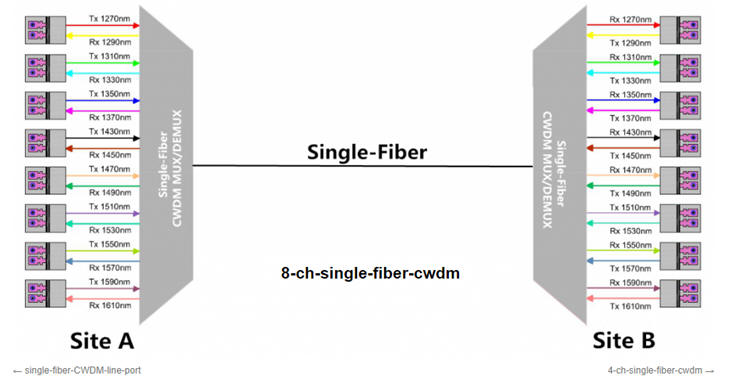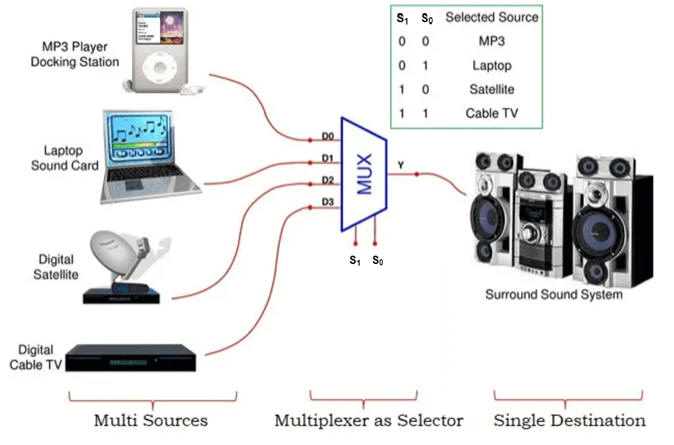Fig. 3. MUX-DeMUX application examples (source: ref. 1, ref.2, ref. 3).

Specifications. Design a MUX_8 using plan_A.

Symbol, truth table, special inputs, example of timing diagram.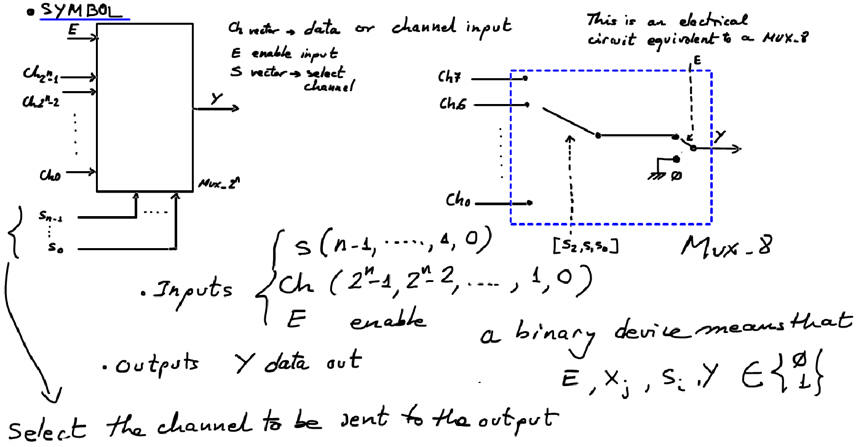Fig. 4. MUX circuit

Truth table example for an 8-channel multiplexer: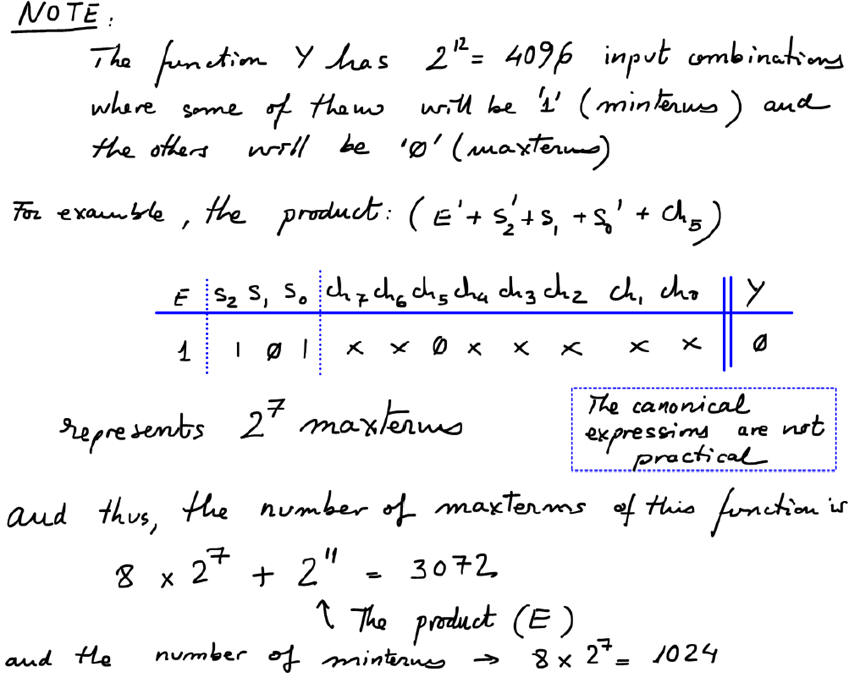Fig. 5. MUX_8 truth table.

Thus, canonical circuits based on maxterms or minterms are not practical.

Fiinally, we can consider a timing diagram to show signals in time.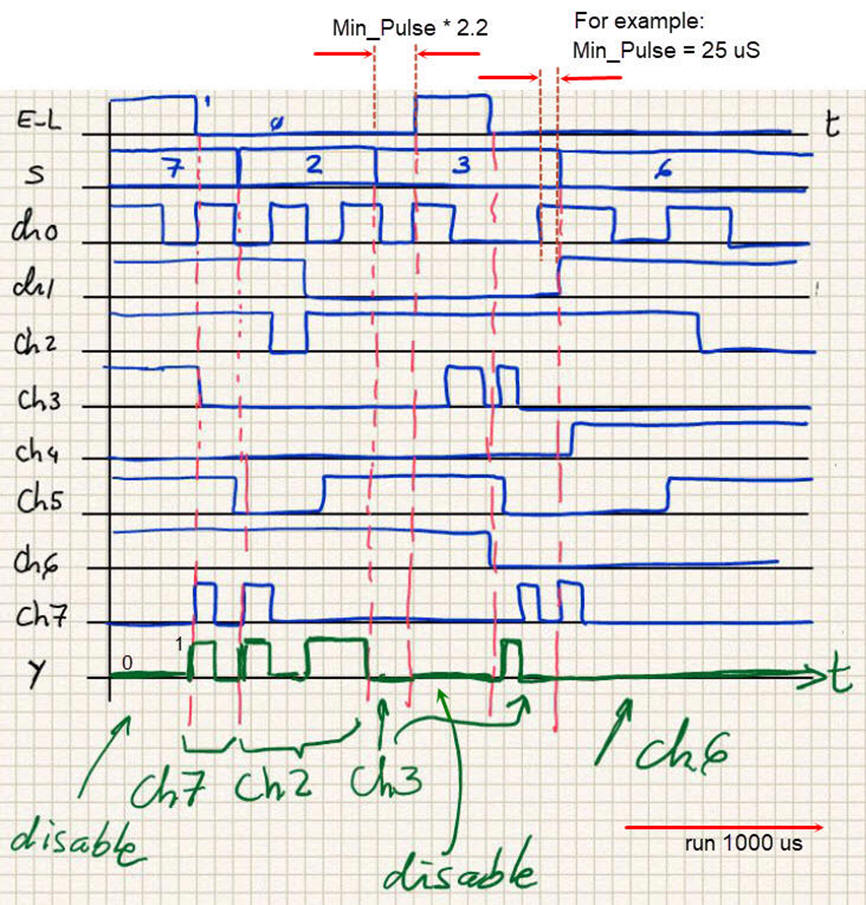Fig. 9. Timing diagram example.

Full planning, development and testing, is presented at LAB2 as design tutorials on plan A and plan B.

In this lesson we will only discuss how we can infer simpler equations like SoP or PoS to be used as plan A. We can simplify algebraic equations inspecting the truth table or using minilog.exe.Fig. 6. Truth table simplified using SoP.

In the same way, we find the dual equation PoS inspecting the truth table or running minilog.exe.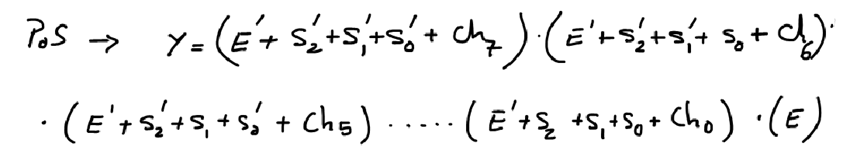Fig. 7. Truth table simplified using PoS.

Additionally, we can "write the circuit" as an algorithm or flowchart that describes its function or behaviour.Fig. 8. High level definition.